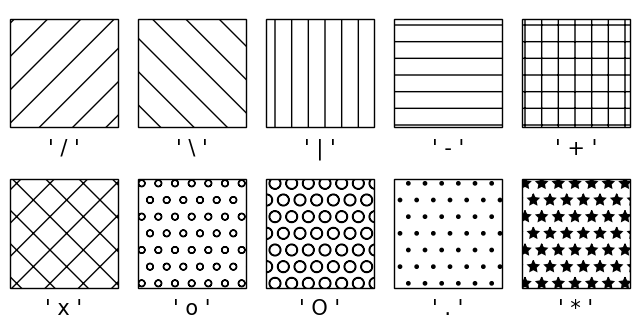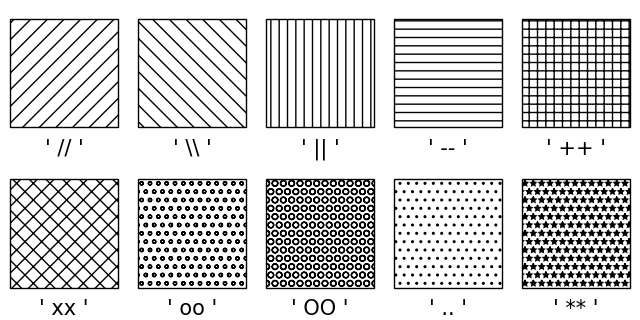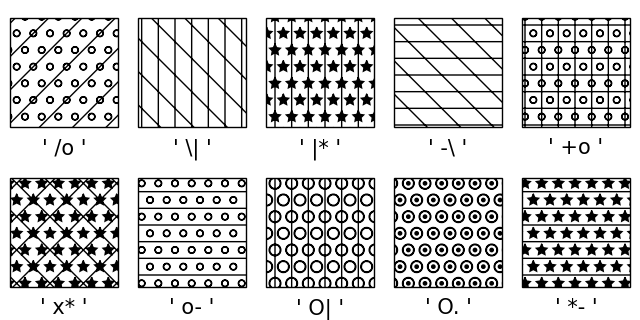# Hatch style reference#

Hatches can be added to most polygons in Matplotlib, including bar, fill_between, contourf, and children of Polygon. They are currently supported in the PS, PDF, SVG, OSX, and Agg backends. The WX and Cairo backends do not currently support hatching.

See also Contourf Hatching for an example using contourf, and Hatch demo for more usage examples.

import matplotlib.pyplot as plt
from matplotlib.patches import Rectangle

fig, axs = plt.subplots(2, 5, layout='constrained', figsize=(6.4, 3.2))

hatches = ['/', '\\', '|', '-', '+', 'x', 'o', 'O', '.', '*']

def hatches_plot(ax, h):
ax.add_patch(Rectangle((0, 0), 2, 2, fill=False, hatch=h))
ax.text(1, -0.5, f"' {h} '", size=15, ha="center")
ax.axis('equal')
ax.axis('off')

for ax, h in zip(axs.flat, hatches):
hatches_plot(ax, h)Hatching patterns can be repeated to increase the density.

fig, axs = plt.subplots(2, 5, layout='constrained', figsize=(6.4, 3.2))

hatches = ['//', '\\\\', '||', '--', '++', 'xx', 'oo', 'OO', '..', '**']

for ax, h in zip(axs.flat, hatches):
hatches_plot(ax, h)Hatching patterns can be combined to create additional patterns.

fig, axs = plt.subplots(2, 5, layout='constrained', figsize=(6.4, 3.2))

hatches = ['/o', '\\|', '|*', '-\\', '+o', 'x*', 'o-', 'O|', 'O.', '*-']

for ax, h in zip(axs.flat, hatches):
hatches_plot(ax, h)References

The use of the following functions, methods, classes and modules is shown in this example:

Total running time of the script: ( 0 minutes 1.232 seconds)

Gallery generated by Sphinx-Gallery